# [Exclusive Bitcoin Blockchain Breakdown] Correcting the Math of Peter Rizun and Craig Wright

The subject of our piece for today’s debunking (April 21st, 2019), is a post that Craig Wright uploaded on the blogging website, Medium.com on November 26th, 2018, titled, ‘Why I troll’.

In specific, it appears that Craig Wright took particular offense to a tweet from Bitcoin ABC developer, Peter Rizun.

Here is a screenshot of the tweet response from Peter Rizun that Craig Wright posted up: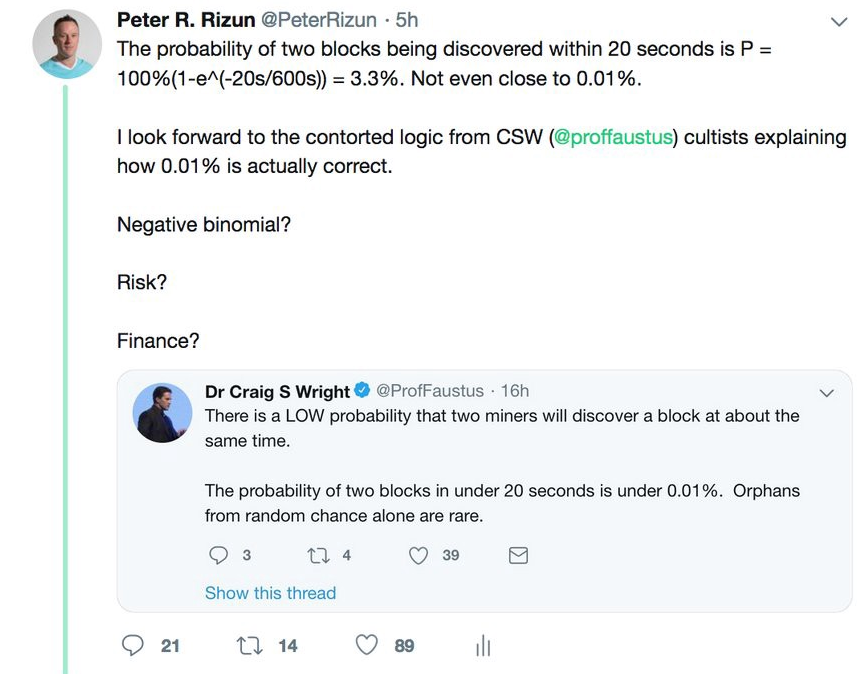### Premise of Craig Wright’s Refutation

Craig Wright begins by stating:

Before we even dig into Craig Wright’s argument, we must first dissect and analyze what it is that Peter and Craig were discussing in the first place.

#### Probability of Block Discovery

Although this should be pretty clear from the screenshot that Craig Wright posted of the conversation between Peter Rizun and himself, we’ll reiterate it for anyone that is lost — Peter and Craig were discussing the probability of orphan blocks occurring.

##### What are Orphan Blocks?

Simply put, on the Bitcoin protocol (and in other PoW currencies), there exists a possibility that two miners find a block at roughly the same time. Since both blocks are technically correct (if the correct PoW has been done), both sets of miners (Miner 1 and Miner 2 we’ll call them) will propagate this information to the network and claim their reward.

This results in some nodes accepting a block from Miner 1 and other nodes accepting a block from Miner 2. In essence the chain has been “split” (although not literally).

Eventually the network decides the ‘winner’, because the block that has the greatest amount of hash power to continue the network will be the version of the blockchain that pervades while the other submitted block that “loses” will essentially be discarded — making it an ‘orphan block’.

##### Craig Wright’s Argument

In his tweet (from the screenshot above), Craig Wright has argued that the chances of such an event happening are 0.01%. In the Medium piece that were are dissecting, Craig Wright actually adjusts his initial calculations to state that the chances of such an event occurring on the blockchain are less than 0.01% by saying, “No, it is not close to 0.01% — it is far less… Sorry, Peter.”

##### Peter Rizun’s Argument

Peter Rizun’s essentially argues that the possibility of an orphan block occurring on the chain is much greater than what Craig Wright has proposed and he puts forth an equation in his refutation to Craig Wright.

When cross-referencing the math provided by Peter Rizun [P=100%(1-e^(-20s/600s))] in Woflram Alpha, a powerful online computational program, it does appear that the math checks out: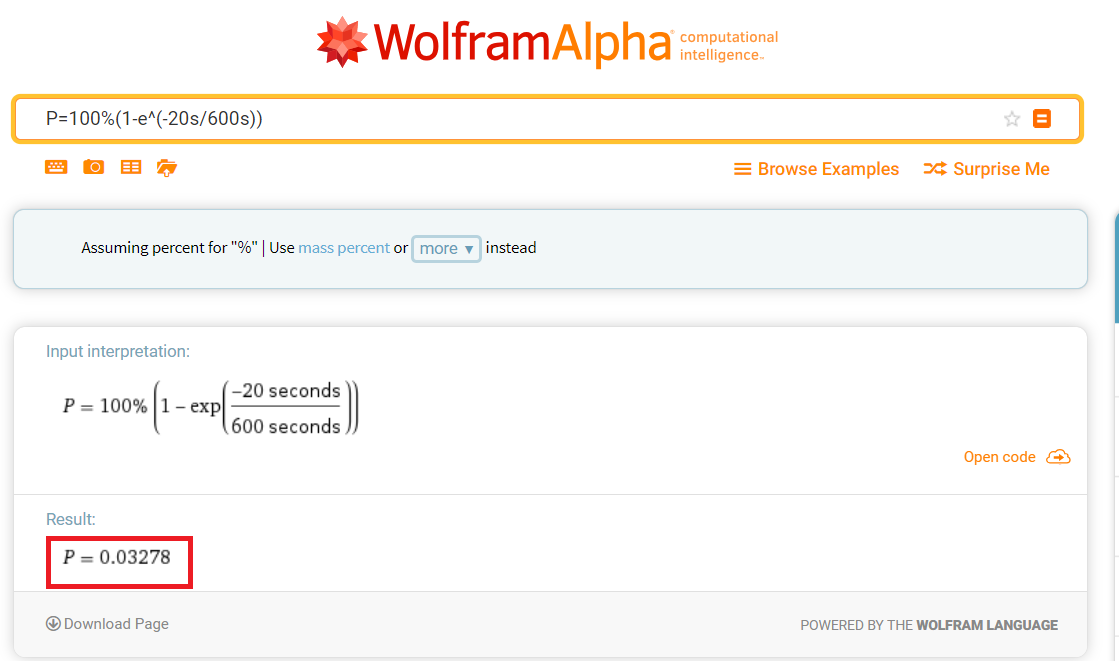### The Math of Both Individuals is Wrong in This Case

Surprisingly, both Peter Rizun and Craig Wright are wrong in their calculations.

Craig Wright does not provide any objective calculations/work for us to assess, so we’ll just keep his answer of 0.01% or less in mind while we examine Peter Rizun’s logic.

#### Flaw in Peter Rizun’s Equation/Logic

One of the central flaws in Peter Rizun’s logic is the use of ‘100%’ in the equation that he puts forth on Twitter in response to Craig Wright:The use of ‘100%’ implies that there is a 100% probability that a block will be found within or at the 10 minute mark.

Even a cursory glance at the blockchain will tell us that this is actually not the case.

In order for us to arrive at the true number, there are a few more numbers that we’re going to have to crunch.

### Figuring Out Blockchain’s Hardest Word Problem

So here is the word problem:

“Assuming that it takes at least 20 seconds for a block to be propagated to the network, what are the chances that an orphan block will be created on the Bitcoin network?”

Despite this question’s appearance of simplicity, there are several steps that must be taken before solving. We’ll go through all of them below before we dissect Craig Wright’s logic.

#### First Objective

In solving this problem, our first objective will be to dissect the actual probability of a block being solved in 10 minutes or less. As stated above, that probability is not 100% and it should not be considered to be such.

As noted in Craig Wright’s response to Peter Rizun, Bitcoin’s retargeting algorithm does utilize a Poisson distribution.

The retargeting formula is as follows: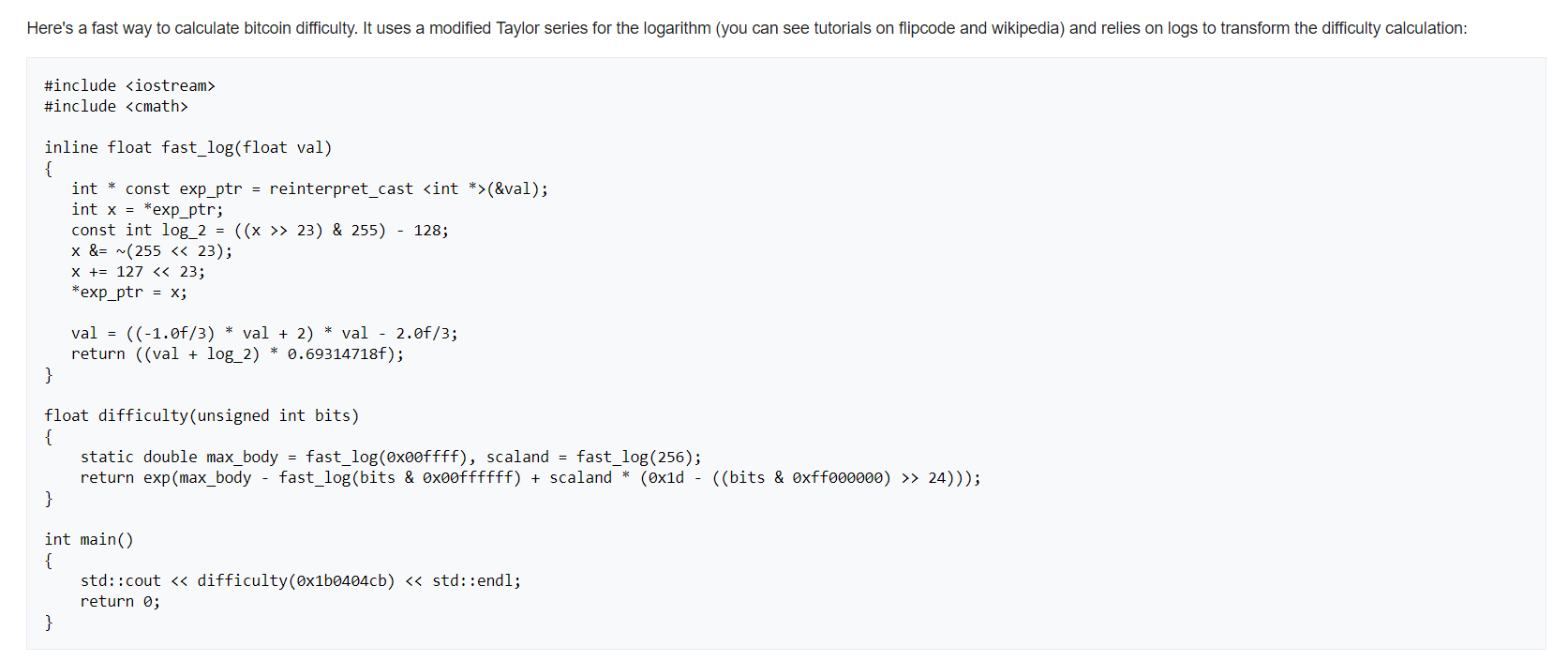Source: https://en.bitcoin.it/wiki/Difficulty#What_is_the_formula_for_difficulty.3F

For coders and math wizards out there, you’ll be able to see from the screenshot above that Bitcoin “target” block creation within 10 minutes based on a probabilistic formula of how long it should take the network to discover the correct nonce value for the next block creation.

#### Viewing the Current Hash Rate

At the time of writing, the current hashrate is estimated to be approximately 42.9 million TH/s (data per blockchain.com):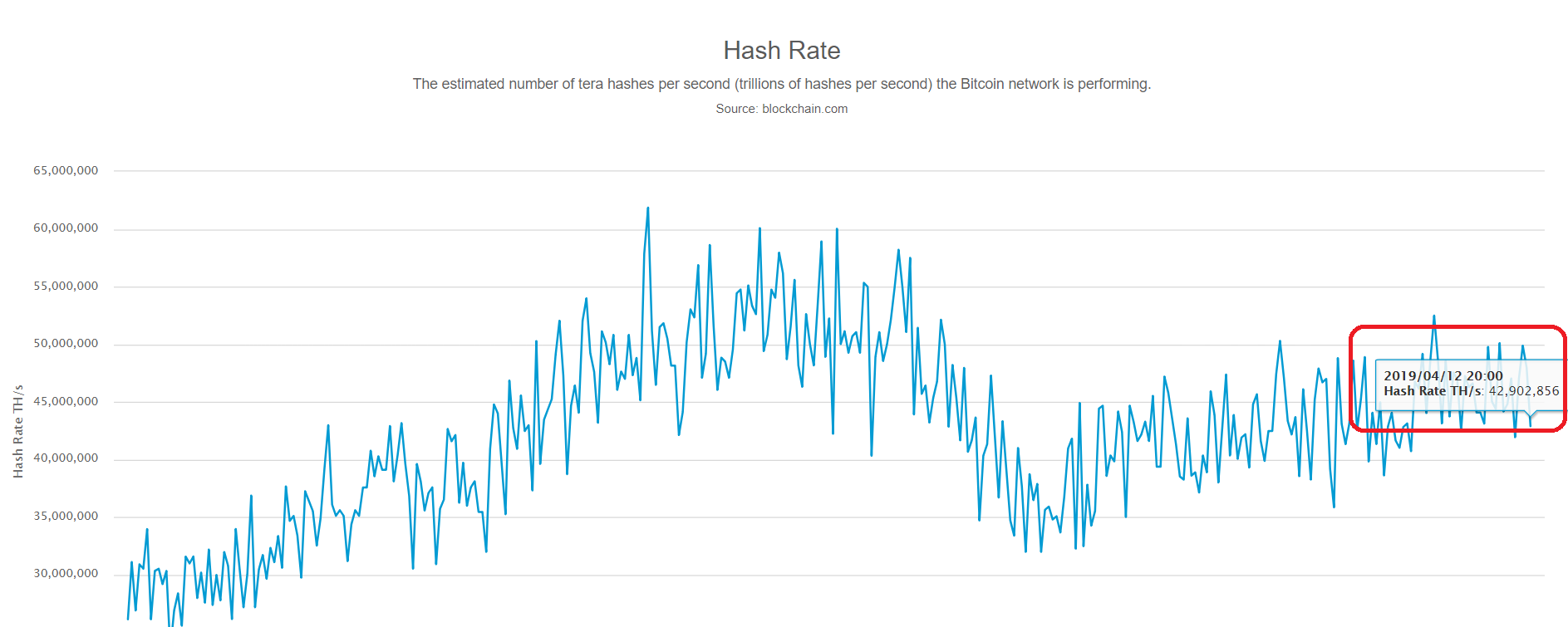Source: https://www.blockchain.com/charts/hash-rate

In order to simplify the metrics here, we will not look at these 42.9 million TH on an individual basis.

Instead, we will borrow a concept from our good friend Charles Hoskinson from Cardano and divide each second into an ‘epoch’ to simplify our process moving forward in the first part of our equation.

Since the Bitcoin blockchain retargets every 2016 blocks by adjusting its difficulty so that it takes, on average, 10 minutes for the entire network to find a solution — we know that there are 600 ‘epochs’ or seconds that we’re looking at here.

So every second worth of trials on the blockchain (currently) = an attempt because we’re looking at the network holisitically and assuming that all miners will continue mining within that 20 second time frame (on the north side of that time frame; between 10 minutes and 10 minutes 20 seconds). Craig argues that this would not be the case, but we’ll get to that part of the argument and debunk it later.

For now, we will continue under the premise that every second represents an opportunity.

#### Breaking Down the Math on the Probability of a Solved Block Within 10 Minutes

So let’s break it down:

n = 620 trials (assuming we set an upper bound limit of 620 seconds, which = 10 minutes 20 seconds)

Probability of success within 600 trials = 1/600.

However, to really calculate this we want the chances of success in a given trial (assuming perfect retargeting and consistent hash rate), which would be (1/600)⁶⁰⁰ (for 600 trials; now you see why we needed to simplify this slightly).

Since these are Bernoulli’s trials (i.e., each second worth of hashes are independent ‘attempts’), we need to use the formula:

X=1-(1-p)^n, where n = # of trials & p = probability of success.

In this case, that leaves us with:

X = 1 — (1–1/600)⁶⁰⁰

The result = .632 (approximately), which means there is a 63.2% chance that we come up with the solution within the 10 minute time frame.

So, p = .632 (per block; each block = 600 seconds)

.632 converts to a 63.2% chance of the network successfully finding the next block.

Now that we have this information, we can solve this riddle once and for all.

### Solving the Equation

Here are the following steps that were undertaken to figure out the solution to the question:

“Assuming that it takes at least 20 seconds for a block to be propagated to the network, what are the chances that an orphan block will be created on the Bitcoin network?”

1. It is already known that traditional targeting should lend a 63.2% chance of the block being found <= 10 minutes (sliding scale up to 63.2% at the height; this is on an exponential distribution).
2. Currently, blockchain.com says that the network hash rate = 42.9M TH/s. We used that for reference in this case.
3. There are 600 seconds in 10 minutes. 600*42.9M = 25.740B TH (total). So there’s a 63.2% chance that 1/25.740B TH will find a successful nonce value at this difficulty.
4. If we divide that success rate (63.2%) by 600 we should get an average success rate of .105% after one second (multiplied cumulatively as we go on; stays consistent w the fact these are all independent trials).
5. Going back to that metric in #1 that there are 42.9M TH/s, we simply divided the .105% by 42.9 to figure out the chances of the first 1 million hashes being successful. This gave us a total of .00244755244%.
6. We did that process in #5 because we’re assuming that a successful nonce find will result in that pool dropping out (everyone else will keep mining because we’re assuming they’re blissfully unaware a solution has been found). Based on pool size, we calculated that would be a 11% drop.
7. 89% of 42.9 = 38.8M. We already calculated the percentage of the first 1M hashes in #5 for this very purpose. Now we can multiply that by 38.8 to get a success rate of .09%/second.
8. From this point, it becomes a classical probability problem like what we used to do in school. Each second is a “trial” (to simplify this so we’re not playing with a dozen+ zeroes). So 20 seconds = 20 trials. We’re looking for 1 success; 19 failures in those 20 trials. Probability of success in each trial = .09% (decimal = .0009).
9. Plugging all of that in gives me a 1.7% chance that a block is found within 20 seconds at that hash rate given the current difficulty.
10. Since these two events must happen in unison, I need to multiply the two probabilities together (63.2% & 1.7%), which gives me a final value of a 1.07% chance.

Below is the intermediate solution for #8: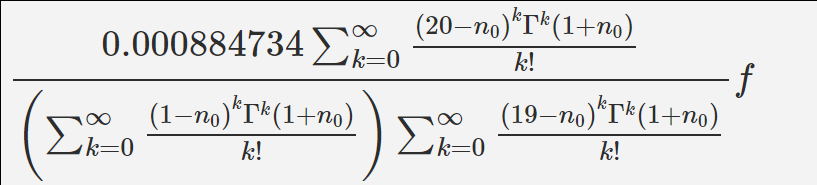### Concluding Remarks on This Formula and the Debate Between Peter Rizun and Craig Wright

The caveat here though is that this is not a +/- 20 second time frame (i.e., the block must be found at exactly 10 minutes in this example and the second one found within 600s — 620s).

If a block is found within, let’s say, 9 minutes, then the probabilities of a second block being found within 20 seconds drops dramatically. If a block is found later than 20 seconds, then the probability of a second block being found within 20 seconds actually decreases as well because the targeting creates a Gaussian distribution.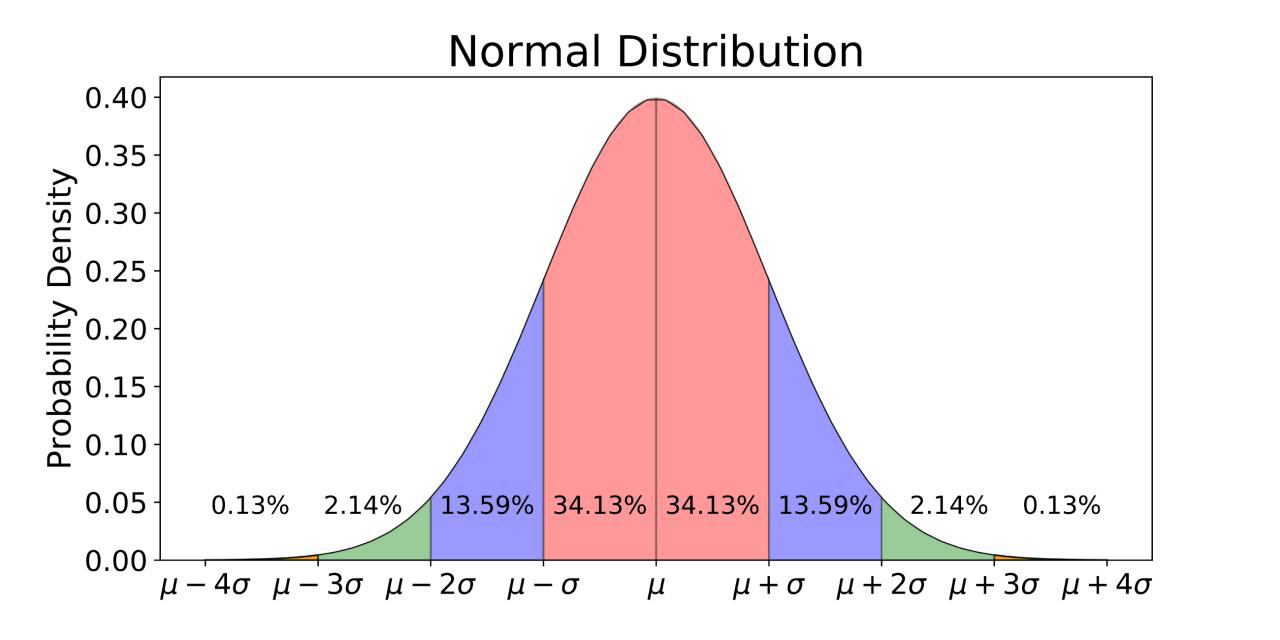Imagine the apex of this distribution = 10 minutes (via targeting). So a 1% chance of this happening is the highest probability on the spectrum. Most of the time it will be less.

### Conclusion

The actual frequency (from what we’ve seen) is between .01%-.1%, but we have to count on mining pools to report that so there’s no telling how accurate those numbers are really as well — but that’s the best we really have to go by.

So Craig Wright is wrong, but not nearly as wrong as Peter Rizun in this case. Readers can take that however they want. This article was not written in defense of Craig Wright or Peter Rizun and certainly not in opposition of either.

However, in the end, it appears that Peter oversimplified this problem and so did Craig.

Enter Best Email to Get Trending Crypto News & Bitcoin Market Updates#### Stay Up to Date!